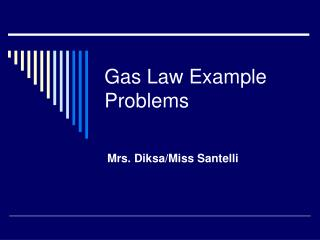Download PresentationGas Law Example ProblemsGas Law Example Problems - PowerPoint PPT Presentation

Download PresentationGas Law Example Problems
An Image/Link below is provided (as is) to download presentation

Download Policy: Content on the Website is provided to you AS IS for your information and personal use and may not be sold / licensed / shared on other websites without getting consent from its author. While downloading, if for some reason you are not able to download a presentation, the publisher may have deleted the file from their server.

- - - - - - - - - - - - - - - - - - - - - - - - - - - E N D - - - - - - - - - - - - - - - - - - - - - - - - - - -
Presentation Transcript

1. Gas Law Example Problems Mrs. Diksa/Miss Santelli

2. The mean molar mass of the atmosphere at the surface of Titan, Saturn’s largest moon, is 28.6 g/mol. The surface temperature is 95K, and the pressure is 1.6 atm. Assuming ideal behavior, calculate the density of Titan’s atmosphere.

3. Dalton’s Law of Partial Pressures What is the total pressure exerted by a mixture of 2.00 g of hydrogen and 8.00 g of nitrogen at 273 K in a 10.0-L vessel?

4. Collecting Over Water Ammonium nitrate, NH4NO2, decomposes upon heating to form N2 gas: NH4NO2→ N2 + 2H2O When a sample of ammonium nitrate is decomposed in a test tube, 511 mL of nitrogen is collected over water at 26 °C and 745 torr total pressure. How many grams of ammonium nitrate decomposed?

5. Effusion and Diffusion • Effusion – the escape of a gas through a tiny hole into an evacuated space. • Diffusion – The spread of one substance throughout a space or throughout a second substance.

6. Notice, did both balloons effuse at the same rate? Speculate why.

7. Why do diffusion rates differ? • While KMT states that the average kinetic energy of the molecules of all gaseous samples is determined by their average temperatures, all gaseous molecules are not the same size. • Recall, KE = ½ mv2 • Because gaseous samples do not all have the same mass, the cannot all have the same root mean square speed, μ = √(3RT/M) • Notice that gases with smaller molar masses will have a greater root mean square speed.

8. Example What is the rms speed of an atom of He at 25°C?

9. Graham’s Law of Effusion • Discovered that effusion rate of a gas is inversely proportional to the square root of its molar mass: • r1/r2 = √(M2/M1) = √[(3RT/M1)/(3RT/M2)]

10. Example • An unknown gas composed of homonuclear diatomic molecules effuses at a rate that is only 0.355 times that of O2 at the same temperature. What is the identity of the unknown?

11. Real Gases • Unlike Ideal Gases which behave under the assumptions of KMT, the molecules of Real Gases • Have finite volumes and • They do attract one another

12. Real Gases • As the pressure of a gaseous sample increases, the volume of the gaseous particles becomes less negligible and, because the molecules are crowded together, intermolecular attraction also increases • As the temperature of a gaseous sample decreases, the average KE of the particles decreases, decreasing the ability of the molecules to overcome the attraction of their neighbors. • Of these two KMT assumptions, intermolecular attraction plays a much bigger role in deviation from ideal behavior.

13. Van der Waals Equation • Johannes van der Waals recognized that for real gases (those under conditions that the ideal gas equation will not all for reasonable predictions), a correction factor would be needed to address the finite volume of the gaseous particles and the intermolecular attractions within the sample.

14. Van der Waals Equation [P + (n2a)/V2](V – nb) = nRT Where nb corrects for the volume of the particles and (n2a)/V2 corrects for molecular attractions. The values of both a and b are van der Waals constants specific to the identity of the gas (see table 10.3 of text)

15. Example Consider a sample of 1.000 mol of CO2 confined to a volume of 3.000L at 0.0°C. Calculate the pressure of the gas using (a) the ideal gas equation and (b) the van der Waals equation.